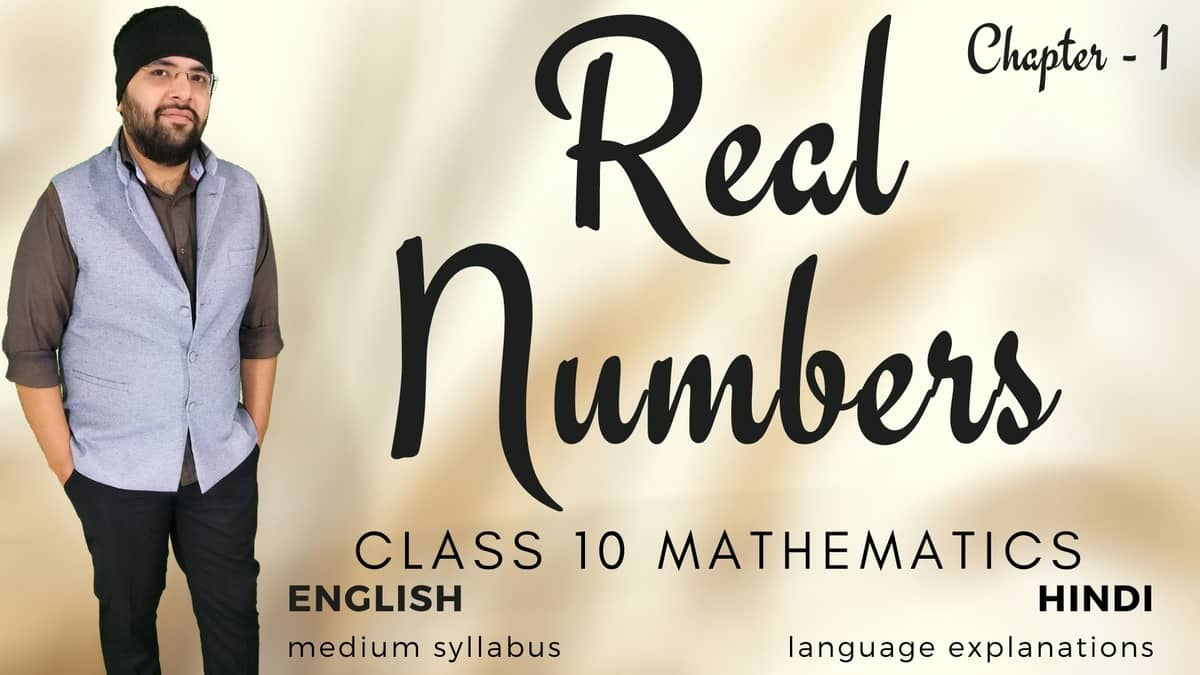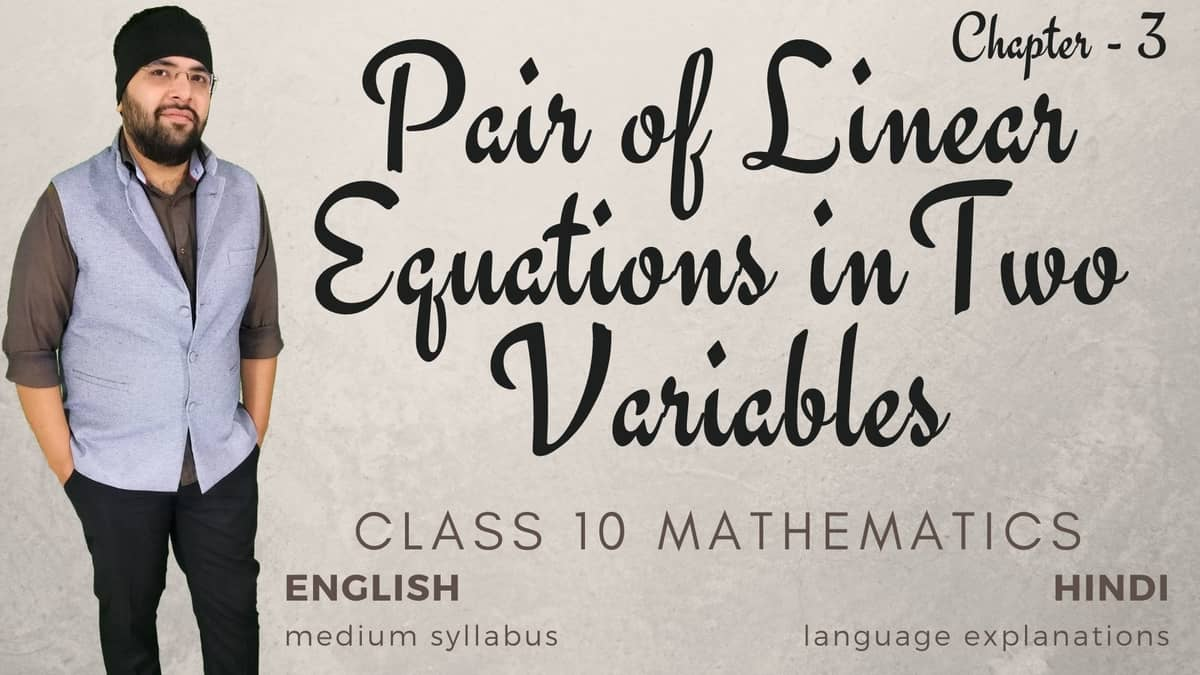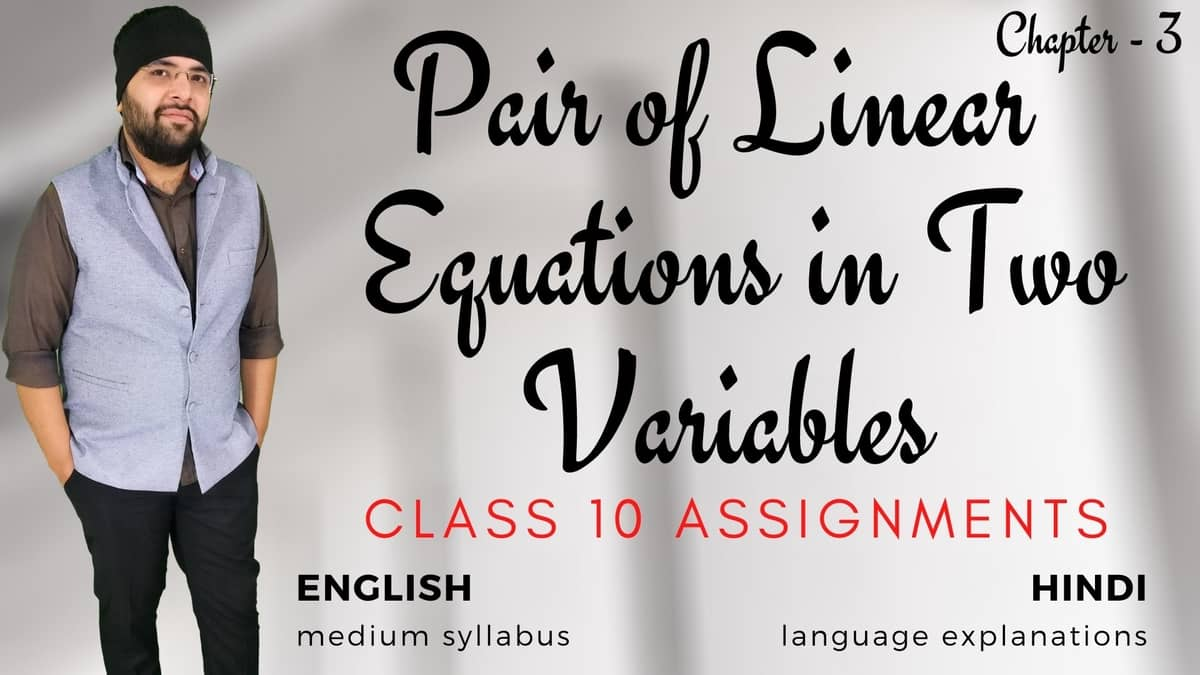# Class 10 Mathematics

The syllabus of Class 10 Mathematics is arranged in the form of courses that can be accessed by the members through this page.

## 1. Real Numbers

12 Months Members Only### Real Numbers Class 10 Maths

Euclid’s division lemma, Fundamental Theorem of Arithmetic - statements after reviewing work done earlier and after illustrating and motivating through examples, Proofs of irrationality, Decimal representation of rational numbers in terms of terminating/non-terminating recurring decimals.

## 3. Pair of Linear Equations in Two Variables

Members Only### Pair of Linear Equations in Two Variables Class 10 Maths

Pair of linear equations in two variables and graphical method of their solution, consistency / inconsistency. Algebraic conditions for number of solutions. Solution of a pair of linear equations in two variables algebraically - by substitution, by elimination and by cross multiplication method. Simple situational problems. Simple problems on equations reducible to linear equations.

Members Only### Pair of Linear Equations in Two Variables Class 10 Assignments

This course is based on the Assignments by Ashish Kumar (Agam Sir). The assignments have questions from NCERT Exemplar, Board's Question Bank, R. D. Sharma etc., under the syllabus of Class 10 Mathematics.

Members Only### Quadratic Equations Class 10 Maths

Standard form of a quadratic equation ax²+bx+c=0, (a ≠ 0). Solutions of quadratic equations (only real roots) by factorization, and by using quadratic formula. Relationship between discriminant and nature of roots. Situational problems based on quadratic equations related to day to day activities to be incorporated.

## 6. Triangles

Members Only### Triangles Class 10 Maths

Definitions, examples, counter examples of similar triangles. 1. (Prove) If a line is drawn parallel to one side of a triangle to intersect the other two sides in distinct points, the other two sides are divided in the same ratio. 2. (Motivate) If a line divides two sides of a triangle in the same ratio, the line is parallel to the third side. 3. (Motivate) If in two triangles, the corresponding angles are equal, their corresponding sides are proportional and the triangles are similar. 4. (Motivate) If the corresponding sides of two triangles are proportional, their corresponding angles are equal and the two triangles are similar. 5. (Motivate) If one angle of a triangle is equal to one angle of another triangle and the sides including these angles are proportional, the two triangles are similar. 6. (Motivate) If a perpendicular is drawn from the vertex of the right angle of a right triangle to the hypotenuse, the triangles on each side of the perpendicular are similar to the whole triangle and to each other. 7. (Prove) The ratio of the areas of two similar triangles is equal to the ratio of the squares of their corresponding sides. 8. (Prove) In a right triangle, the square on the hypotenuse is equal to the sum of the squares on the other two sides. 9. (Prove) In a triangle, if the square on one side is equal to sum of the squares on the other two sides, the angles opposite to the first side is a right angle.

## 8. Introduction to Trigonometry

12 Months Members Only### Introduction to Trigonometry Class 10 Maths

Trigonometric ratios of an acute angle of a right-angled triangle. Proof of their existence (well defined); motivate the ratios whichever are defined at 0°, 90°. Values of the trigonometric ratios of 30°, 45° and 60°. Relationships between the ratios. Proof and applications of the identity sin²A + cos²A = 1. Only simple identities to be given. Trigonometric ratios of complementary angles.

## 12. Area Related to Circles

Members Only### Area related to Circles Class 10 Maths

Motivate the area of a circle; area of sectors and segments of a circle. Problems based on areas and perimeter / circumference of the above said plane figures. (In calculating area of segment of a circle, problems should be restricted to central angle of 60°, 90° and 120° only. Plane figures involving triangles, simple quadrilaterals and circle should be taken.)

## 13. Surface Areas and Volumes

Members Only### Surface Areas and Volumes Class 10 Maths

1. Surface areas and volumes of combinations of any two of the following: cubes, cuboids, spheres, hemispheres and right circular cylinders/cones. Frustum of a cone. 2. Problems involving converting one type of metallic solid into another and other mixed problems. (Problems with combination of not more than two different solids be taken).